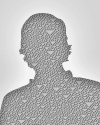#Wolfram Summer School

# Alumni# Kevin James

Summer School

Class of 2015

## Project: Inputting Data with Neural Networks

We attempt to generate cat images by first passing a training set of cat images through a trained neural network and modeling the distribution of the images in the neural network feature space. The feature space aids our models in two ways: there are few dimensions (in our case 1024), and, in the process of classifying the images, the neural network segregates members of each class in space. We modeled the distribution of cat images in this neural network feature space. We used this model to try filling in small pieces of missing information.

The basic idea is to use an image-classifying neural network to generate new instances of a class. Suppose we look at the set of all cat pictures. In theory, with enough image samples, we could generate a model that describes the pixel distribution of cat images, and sample a new image. The main problem with this approach is that sample space for just a 256 x 256 x 3 image has over 100 k dimensions, and given the variance of cat images, adequately sampling the space is impractical.

Our approach is to first run the images through a neural network, and sample the images at some point within the neural network (at first this will be in the last layer before output). Because the neural network reduces the dimensions of the space (in our case to around 1,000 dimensions), we can better sample it and come up with a more appropriate model.

Once we have a model, we need an image that samples from the generated distribution. This can be done via the MCMC (Monte Carlo Markov chain) Metropolis algorithm. In it, you start with an initial image (likely random), I0. You then create I1 via a random mutation on I0 (manipulate a pixel). Then you run the images through the neural network, get the output at the layer you are operating at, and determine the probabilities of each in the model. You then accept the mutation with probability P(I1) P(I0). After enough iterations, this will converge to a random sample from the distribution.

The steps from my project are as follows:

1. Get trained neural network (done)
2. Get cat images (have 1.4 k so far)
3. Run through neural network to sample space (using last layer at first)
4. Generate model for samples (will start with multivariate Gaussian, compare to Restricted Boltzmann Machine if time permits)
5. Write MCMC to generate new image
6. Compare models (which layer to sample from, which model to use) by running test images through and comparing log-likelihood of the test images (want to maximize this)## Wolfram Summer School | Champaign, IL, USA | July 3 29–July 22, 2022

APPLY NOW
• 21st Annual Wolfram Summer School
• Bentley University, Waltham, MA, USA
• June 25–July 15, 2023
• Apply now